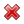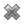# Fight Finance

#### CoursesTagsRandomAllRecentScoresScoreskeithphw $6,011.61 Jade$1,815.80 Chu $789.98 royal ne...$750.00 Leehy $713.33 Visitor$650.00 ZOE HY $640.00 JennyLI$625.61 Visitor $590.00 Visitor$555.33 Visitor $550.00 Visitor$550.00 Visitor $540.00 Visitor$500.00 Yizhou $489.18 Visitor$480.00 Visitor $470.00 Visitor$464.70 Visitor $460.00 Visitor$460.00

You want to buy an apartment priced at $300,000. You have saved a deposit of$30,000. The bank has agreed to lend you the $270,000 as a fully amortising loan with a term of 25 years. The interest rate is 12% pa and is not expected to change. What will be your monthly payments? Remember that mortgage loan payments are paid in arrears (at the end of the month). You just signed up for a 30 year fully amortising mortgage loan with monthly payments of$1,500 per month. The interest rate is 9% pa which is not expected to change.

To your surprise, you can actually afford to pay $2,000 per month and your mortgage allows early repayments without fees. If you maintain these higher monthly payments, how long will it take to pay off your mortgage? Find the sample standard deviation of returns using the data in the table:  Stock Returns Year Return pa 2008 0.3 2009 0.02 2010 -0.2 2011 0.4 The returns above and standard deviations below are given in decimal form. A mature firm has constant expected future earnings and dividends. Both amounts are equal. So earnings and dividends are expected to be equal and unchanging. Which of the following statements is NOT correct? A stock is expected to pay a dividend of$5 per share in 1 month and $5 again in 7 months. The stock price is$100, and the risk-free rate of interest is 10% per annum with continuous compounding. The yield curve is flat. Assume that investors are risk-neutral.

An investor has just taken a short position in a one year forward contract on the stock.

Find the forward price $(F_1)$ and value of the contract $(V_0)$ initially. Also find the value of the short futures contract in 6 months $(V_\text{0.5, SF})$ if the stock price fell to \$90.

Which of the below formulas gives the payoff at maturity $(f_T)$ from being short a future? Let the underlying asset price at maturity be $S_T$ and the locked-in futures price be $K_T$.

Question 785  fixed for floating interest rate swap, non-intermediated swap

The below table summarises the borrowing costs confronting two companies A and B.

 Bond Market Yields Fixed Yield to Maturity (%pa) Floating Yield (%pa) Firm A 3 L - 0.4 Firm B 5 L + 1

Firm A wishes to borrow at a floating rate and Firm B wishes to borrow at a fixed rate. Design a non-intermediated swap that benefits firm A only. What will be the swap rate?

Question 903  option, Black-Scholes-Merton option pricing, option on stock index

A six month European-style call option on the S&P500 stock index has a strike price of 2800 points.

The underlying S&P500 stock index currently trades at 2700 points, has a continuously compounded dividend yield of 2% pa and a standard deviation of continuously compounded returns of 25% pa.

The risk-free interest rate is 5% pa continuously compounded.

Use the Black-Scholes-Merton formula to calculate the option price. The call option price now is:

Question 904  option, Black-Scholes-Merton option pricing, option on future on stock index

A six month European-style call option on six month S&P500 index futures has a strike price of 2800 points.

The six month futures price on the S&P500 index is currently at 2740.805274 points. The futures underlie the call option.

The S&P500 stock index currently trades at 2700 points. The stock index underlies the futures. The stock index's standard deviation of continuously compounded returns is 25% pa.

The risk-free interest rate is 5% pa continuously compounded.

Use the Black-Scholes-Merton formula to calculate the option price. The call option price now is:

You work for XYZ company and you’ve been asked to evaluate a new project which has double the systematic risk of the company’s other projects.

You use the Capital Asset Pricing Model (CAPM) formula and input the treasury yield $(r_f )$, market risk premium $(r_m-r_f )$ and the company’s asset beta risk factor $(\beta_{XYZ} )$ into the CAPM formula which outputs a return.

This return that you’ve just found is: